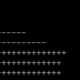# XML/Postgresql Rendering

 0 Heyho! I try to render my map from a Postgresql/PostGIS Database. I use a simple XML-Stylesheet. Can somebody please tell me, what this error-message means and how I can fix it? That would be really kind! The error-massage is quoted at the end of this post. If needed, I can quote/upload my code as well. Greetings Matze ``````Traceback (most recent call last): File "C:/0A/run_python", line 3, in execfile(r"C:\0A\7\schwentine.py") File "C:\0A\7\schwentine.py", line 57, in render_image() File "C:\0A\7\schwentine.py", line 21, in render_image mapnik.load_map(m,r"C:\0A\7\schwentine.xml") RuntimeError: Postgis Plugin: PSQL error: ERROR: syntax error at or near "planet_osm_line" LINE 1: ... srid FROM geometry_columns WHERE f_table_name=' 'planet_osm... ^ Full sql was: 'SELECT f_geometry_column, srid FROM geometry_columns WHERE f_table_name=' 'planet_osm_line' '' (encountered during parsing of layer 'tutorial' in map 'C:\0A\7\schwentine.xml') `````` asked 22 Mar '12, 08:19MHein 141●11●11●16 accept rate: 0%

 1 What is the last line of your inc/settings.xml.inc file? If you are running the default setup it should be It looks like you might have put in extra quotes. answered 23 Mar '12, 06:48pnorman 2.4k●5●21●40 accept rate: 18% This is my XML-Stylesheet: `````` ]> <Style name="world"></Style> <Style name="coast-poly"></Style> <Style name="builtup"></Style> <Layer name="world"></Layer> <Layer name="coast-poly"></Layer> <Layer name="builtup"></Layer> <Layer name="tutorial"> <StyleName>tutorial-fill</StyleName> <Datasource> <Parameter name="type">&dbtype;</Parameter> <Parameter name="user">&dbuser;</Parameter> <Parameter name="dbname">&dbname;</Parameter> <Parameter name="table"> 'planet_osm_points' </Parameter> <Parameter name="estimate_extent">false</Parameter> <Parameter name="extent">-20037508,-19929239,20037508,19929239</Parameter> </Datasource> </Layer> `````` `````` (23 Mar '12, 06:53) MHein This is my Python-file `````` #!/usr/bin/python import mapnik from mapnik import Layer, PostGIS import sys, os def render_image(): ll = (-180.0,-90.0, 180.0,90.0) prj = mapnik.Projection("+proj=utm +zone=32U +ellps=GRS80 +datum=WGS84 +units=m +no_defs") #prj = mapnik.Projection("+proj=tmerc +lat_0=49 +lon_0=-2 +k=0.999601 +x_0=400000 +y_0=-100000 +ellps=airy +units=m +towgs84=446.448,-125.157,542.060,0.1502,0.2470,0.8421,-20.4894 +units=m +nodefs") #prj = mapnik.Projection("+proj=latlong +datum=WGS84") c0 = prj.forward(mapnik.Coord(ll,ll)) c1 = prj.forward(mapnik.Coord(ll,ll)) z = 3 imgx = 336 * z imgy = 450 * z m = mapnik.Map(imgx,imgy) mapnik.load_map(m,r"C:\0A\7\schwentine.xml") db_params = dict( dbname = 'gis_schwentine', user = 'Hein', password = '1234', host = 'localhost', port = 5432, estimate_extent = False, extent = "3390650.221286806, -3163145.87245787, 3609898.596229789, -2956043.104540316" ) lyr = Layer('points',"+init=epsg:900913") db_params['table'] = (planet_osm_point) lyr.datasource = PostGIS(**db_params) lyr.styles.append('points') m.layers.append(lyr) if hasattr(mapnik,'mapnik_version') and mapnik.mapnik_version() >= 800: bbox = mapnik.Box2d(c0.x,c0.y,c1.x,c1.y) else: bbox = mapnik.Envelope(c0.x,c0.y,c1.x,c1.y) m.zoom_to_box(bbox) im = mapnik.Image(imgx,imgy) mapnik.render(m, im) view = im.view(0,0,imgx,imgy) # x,y,width,height view.save("schwentine.png",'png') ``````if name == "main": render_image() `````` (23 Mar '12, 07:41) MHein
 toggle preview community wiki:

By Email:

Markdown Basics

• *italic* or _italic_
• **bold** or __bold__
• image?![alt text](/path/img.jpg "title")
• numbered list: 1. Foo 2. Bar
• to add a line break simply add two spaces to where you would like the new line to be.
• basic HTML tags are also supported

Question tags:

×165
×134
×115
×84
×21

question asked: 22 Mar '12, 08:19

question was seen: 5,536 times

last updated: 23 Mar '12, 08:28